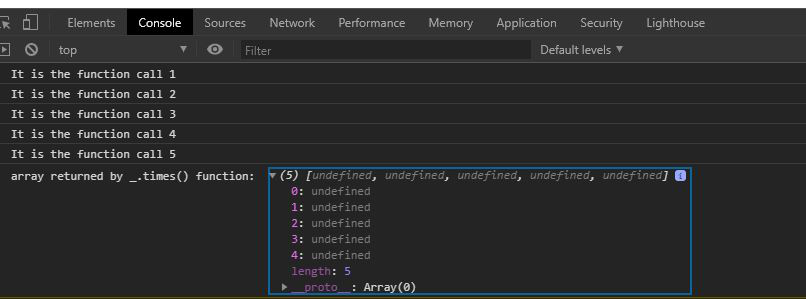Open In App
Related Articles

# Underscore.js _.times() Function

Underscore.js is a JavaScript library that makes operations on arrays, string, objects much easier and handy.
The _.times() function in underscore.js is used to call the function a particular number of times i.e. execution of a function(f) “n” number of times.

Note: It is very necessary to link the underscore CDN before going and using underscore functions in the browser. When linking the underscore.js CDN link, the “_” is attached to the browser as a global variable.

Syntax:

`_.times(n, iteratee)`

Parameters:It takes following parameters:

• n: It tells how many times a function is needed to be executed.
• iteratee : It is a function that is to be invoked n times.

Return Value: It produces an array of returned values and this array is returned by the function.

Example 1:

## HTML

 ```<``html``>` `<``head``>``    ``<``script` `src``=``"https://cdnjs.cloudflare.com/ajax/libs/underscore.js/1.9.1/underscore-min.js"``>``    ````` `<``body``>``    ``<``script``>``        ``let n = 5``        ``let func = () => {``            ``console.log(`This function``                ``is called \${n} times \n`)``        ``}` `        ``// The _.times function executes``        ``// the above func function n times``        ``_.times(n, func);``    ````` ``

Output:Example 2:

## Javascript

 ```` ```    ````` ```    ````` ``

Output: